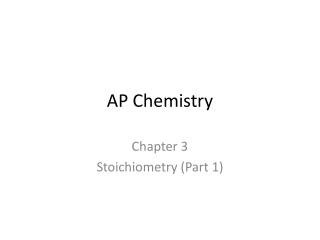DownloadDownload PresentationAP Chemistry

# AP Chemistry

Télécharger la présentation## AP Chemistry

- - - - - - - - - - - - - - - - - - - - - - - - - - - E N D - - - - - - - - - - - - - - - - - - - - - - - - - - -
##### Presentation Transcript

1. AP Chemistry Chapter 3 Stoichiometry (Part 1)

2. 3.1 Atomic Masses (p. 80) An atomic mass unit (amu) is defined such that the mass of 12C is exactly 12 amu. Atomic mass in the periodic table is the average atomic mass of all stable isotopes of the element. Problem: What is the atomic mass of Helium? (4.003 amu) Problem: Why is the atomic mass Tc (technetium) in parentheses? (estimated mass of artificial element) Problem: Chlorine has two stable isotopes. The mass of one isotope is 34.97 amu. Its relative abundance is 75.53%. What is the mass of the other isotope? (34.97 amu)(0.7553) + x(0.2447) = 35.45 amu x = 36.94 amu Problem: Gallium has two stable isotopes of masses 68.93 amu (69Ga) and 70.92 amu (71Ga). What are the relative abundances of the two isotopes? The average atomic mass of Gallium is 69.72 amu. x + y = 1.000 68.93x + 70.92y = 69.72 x = 0.6030 (60.3%) y = 0.397 (39.7%)

3. 3.2 The Mole (p. 83)mole-number equal to the number of carbon-atoms in exactly 12 grams of pure 12C. Called Avogadro’s number (6.022 x 1023) to honor his contribution to chemistry. 6.022 x 1023amu = 1 g Therefore, 1 amu = ? g = __________________ g Problem: convert 12.04 x 1023 atoms He to moles 12.04 x 1023 atoms x (1 mol/6.02 x 1023 atoms) = 2 mol He Problem: convert 5000. grams K to moles 5000. g K x (1 mol K/39.10 g) = 127.87 = 127.9 mol K Problem: convert 5.00 mol Ag to mass in grams 5.00 mol Ag x (107.9 g Ag/1 mol Ag) = 539.5 = 540. g

4. Problem: convert 25.0 mol Ar to number of atoms 25.0 mol Ar x (1 mol/6.02 x 1023 atoms) = 1.505 x 1025 atoms Problem: Convert 3.01 x 1023 atoms Ni to mass in grams 3.01 x 1023 atoms Ni x (1 mol/6.02 x 1023 atoms) x (58.69 g/ 1 mol Ni) = 29.345 = 29.3 g Problem: convert 2000. grams of Mg to number of atoms 2000. g Mg x (1 mol/24.3 g) x (6.02 x 1023 atoms) = 4.95 x 1025 atoms Mg

5. 3.3 Molar Mass (p. 87)- the mass in grams of 1 mole of a compound Problem: calculate the molar mass of Na2SO4 2(22.9) + 32.06 + 4(16.00) = Problem: calculate the molar mass of copper (II) sulfate pentahydrate. 63.5 + 32.06 + 4(16.00) + 5(18.00) = Problem: convert 72 grams HCl to number of molecules 72 g HCl x (1 mol/36.5 g) x (6,02 x 1023 molecules/1 mol) = 1.204 x 1024 molecules Problem: convert 1.81 x 1024 molecules H2S to mass in grams 1.81 x 1024 molecules x (1 mol/6.02 x 1023 molecules) x (34 g/1 mol) = 102.2 = 102 g

6. 3.4 Percent composition (p. 91) Use the molar mass of a compound to determine the % composition by mass of the compound. (Also, be able to do in reverse.) Problem: determine the % composition of Na2SO4 (molar mass =

7. 3.5 Determining the Formula of a compound (p. 93) • Empirical formula determination: (p. 99) • Determine the # of moles of each element present in the compound • Determine the relative # of moles of each element by dividing the smallest # of moles into all of the others • If you do not get small whole numbers, then multiply by each number by an integer. (The small whole numbers represent subscripts)

8. Molecular Formula Determination: (p. 99-100) • (same steps as empirical formula determination) • Calculate the molar mass of the empirical formula. • Compare it to the given or known molar mass of the compound. • If it is the same, then the EF = MF; if not, it must be a multiple (again, some small whole number) of the molar mass of the empirical formula. • Multiple the EF by the small whole number to get the MF.

9. Problem: find the empirical formula of a compound with 1.645 g N & 0.355 g H

10. Problem: Find the empirical formula of a compound with 20.23% Al and 79.77% Cl. (Hint: assume 100 grams)

11. Problem: A compound of phosphorus and oxygen contains 56.36% oxygen. Its molar mass is 283.9 g. What is the molecular formula? (determine empirical formula first)